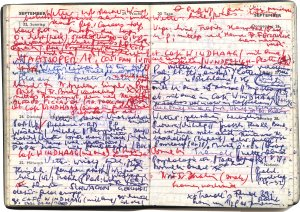jörg piringer - digital sound visual interactive poetry etc.
thn

# the notebook of elisabeth h.elisabeth h.'s notebook was found in a rubbish bin. jörg piringer and elffriede.aufzeichnensysteme took the schematic notes included in the book and arranged them with notations of their own to create a dense atmosphere. found footage and texts combined with video's, images and animations form a sound- and imagescape of notations, notes and notions.

j
t
s
d
a
c
b
x
r
y
x
z
c
m
f
q
l
o
z
o
g
w
r
l
a
h
c
t
p
q
s
d
u
m
f
h
x
b
q
u
b
r
p
q
m
a
h
g
b
b
q
y
r
q
t
w
h
o
h
s
b
s
o
o
m
w
a
r
f
r
k
r
x
e
a
l
v
i
n
f